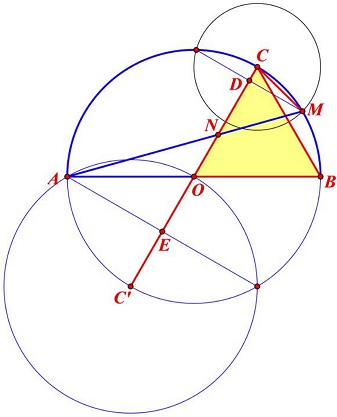# Another Golden Ratio in Semicircle

Bùi Quang Tuån has posted on the CutTheKnotMath facebook page a simple construction of the golden ratio. Below is a trigonometric proof. Proof 2 is the original with Bùi Quang Tuån.

### Construction

In a semicircle $(O)$ with a diameter $AB,$ let $OBC$ be an equilateral triangle with $C$ on $(O);$ $M$ on $(O)$ with $CM=\frac{1}{2}BC.$ Define $N$ as the intersection of $AM$ and $CO.$Then $\displaystyle\frac{CN}{NO}=\phi,$ the golden ratio.

### Proof 1

Let the angles $\alpha,$ $\beta$ be defined as indicated below and assume the radius of the semicircle to be $1.$From $\Delta OCM,$ $\displaystyle\sin\alpha =\frac{1}{4},$ implying $\displaystyle\cos\alpha =\frac{\sqrt{15}}{4}.$

By the Cosine Law in $\Delta BCM,$ $BM^{2}=BC^{2}+BM^{2}-2BC\cdot BM\cos\alpha,$ from which $\displaystyle BM=\frac{\sqrt{15}-\sqrt{3}}{4}.$ By the Law of Sines, $\displaystyle\sin\beta=\frac{\sqrt{15}-\sqrt{3}}{8}.$ It can be verified that $\displaystyle\cos\beta=\frac{3\sqrt{15}+1}{8}.$

Apply now the Law of Sines in $\Delta ANO:$ $\displaystyle NO=\frac{\sin\beta}{\sin(60^{\circ}-\beta)}.$ By the addition formula,

\begin{align}\displaystyle \sin(60^{\circ}-\beta ) &=\sin 60^{\circ}\cos\beta-\cos 60^{\circ}\sin\beta\\ &= \frac{\sqrt{3}}{2}\cdot\frac{3\sqrt{15}+1}{8}-\frac{1}{2}\cdot \frac{\sqrt{15}-\sqrt{3}}{8}\\ &= \frac{\sqrt{15}+\sqrt{3}}{8}. \end{align}

It follows that

$\displaystyle NO=\frac{\sin\beta}{\sin(60^{\circ}-\beta)}=\frac{\sqrt{15}-\sqrt{3}}{\sqrt{15}+\sqrt{3}}=\frac{3-\sqrt{5}}{2}.$

Thus $\displaystyle CN=1-NO=\frac{\sqrt{5}-1}{2}$ and $\displaystyle \frac{CN}{NO}=\phi,$ as required.

### Proof 2

Two right triangles $CDM\;$ and $CMC'\;$ are similar therefor: $\displaystyle\frac{CD}{CM} = \frac{CM}{CC'} = \frac{1}{4}\;$ and $E\;$ is midpoint of $OC'.$From this we can calculate all segments in the configuration by radius of semicircle and at the end, get the golden ratio as needed.### Golden Ratio